• 0

# Box Plot

Go to solution Solved by Amlan Dutt,

Box Plot

Box Plot or Box and Whisker Plot is a graphical representation tool for continuous data set. It visually depicts the central tendency (in the form of median) and the spread (in terms of Inter-Quartile Range) of the data set.

An application-oriented question on the topic along with responses can be seen below. The best answer was provided by Amlan Dutta, Mohamed Asif & Natwar Lal.

Applause for the respondents -Amlan Dutta, Kiran Kumar, Mohamed Asif , Vastupal Vashisth, Nilesh Akre, Natwar Lal, Guruprasad R, Rachit Vohra, Sreyash Sangam

Also review the answer provided by Mr Venugopal R, Benchmark Six Sigma's in-house expert.

## Question

Q﻿﻿. 172  Box Plot provides a graphical summary of data. What are the specific benefits/ insights obtained by using a Box Plot. Explain with examples

Note for website visitors - Two questions are asked every week on this platform. One on Tuesday and the other on Friday.

## Recommended Posts

• 1
• Solution

Check this one out! A picture worth thousand words. Box plot comic

So the benefits are obvious.

• Box plots are easy to interpret.
• It is five point summary (Q1, Q2 ,Q3,Upper and Lower Fences)
• It handles huge uni-variate observations pretty quick; because median doesn't require an analytic solution unlike mean
• It handles non normal distribution pretty well

Let's see at anatomy of box plots.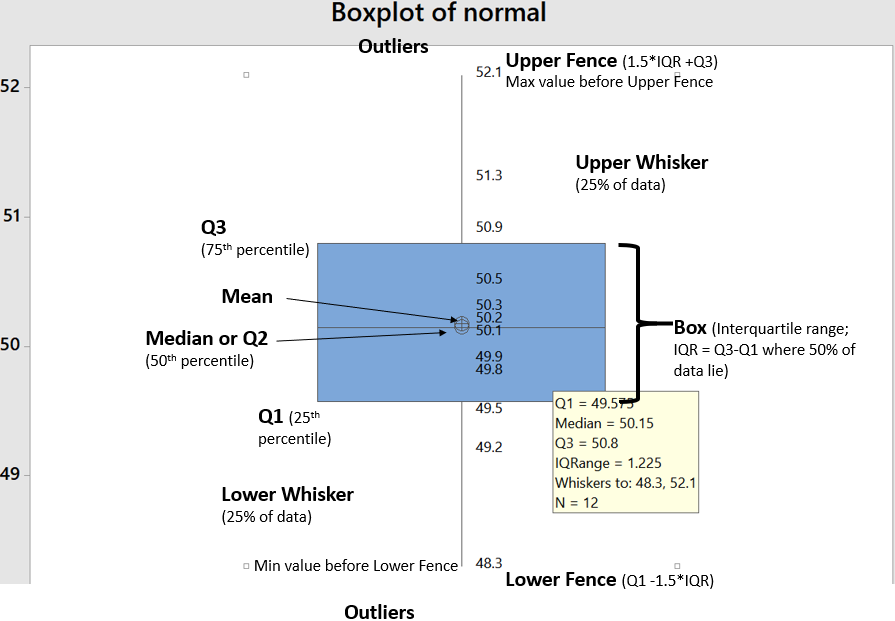So It helps visualizing 25th, 50th, 75th percentiles along with mean and outliers. Box part of it contains 50% of data while remaining 50% lies with both whiskers collectively (...along with outliers). One question arise that why did John Tukey (inventor of box plot) selected 1.5 times IQR?

Well because the tendency really is to consider most distributions to be Gaussian by reflex, and for a Gaussian distribution, it works well.
What I mean is, for a nice Gaussian distribution, (with s as Std. Dev and X as Mean)

• Q1 = -s0.67 + X, and Q3 = s0.67 + X,  (from the property of standard scores for a Gaussian distribution)
• IQR would be Q3-Q1, So IQR = 1.34s  (from above)
• Tukey’s lower bound = Q1-1.5IQR = 1.34s , which is X-2.7s

If you chose Q1-1IQR, it would be X-2s (too many outliers), and if you chose Q1-2IQR, it would be 4s (too few outliers)
1.5IQR allows just under 3s, (and is easier to handle than 1.567IQR). In other words, if +-3s gives ~.3% observations as outliers, box plots with +-2.7s will give .7% observations as outliers.

It handles mildly non-normal distribution well showing skewness. Below are examples of left skewed, no skew and right skewed data from top to bottom. Notice the differences in respective box plots.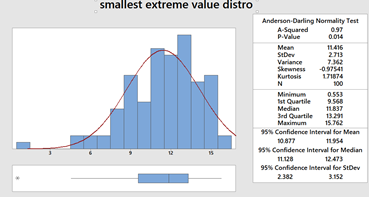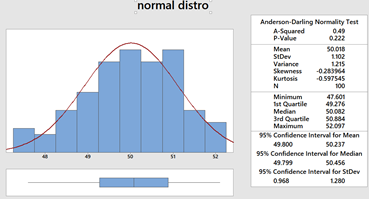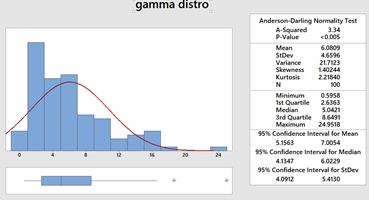Another interesting fact is there are nothing like Q0 and Q4. Why?

Try finding 0th or 100th percentile of any uni-variate data. These are undefined! reason being percentiles are calculated from CDF by popular softwares while assuming normal distribution by default. We already know that CDF of normal distribution is unbounded. That's why for highly skewed distributions box plots are not good choice. Some softwares call Q0 and Q4 as minimum and maximum but then they no more remain fences of whiskers because there can be observations as outliers.

Notably box plots are poor with representing "holes", below is a box plot with mixture of two normal distributions (mean of 50 and 90; same SD of 1). Although there are no observations between 53 and 88 yet the box misrepresents it (...or it increases chances on misinterpretation).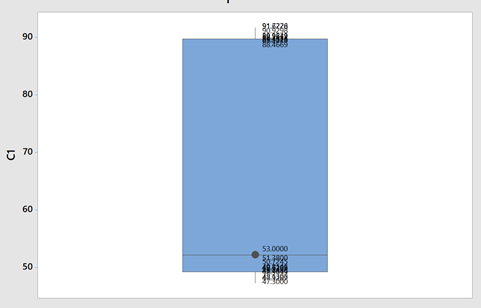Another variant of box plot is with notches which give 95% CI for median.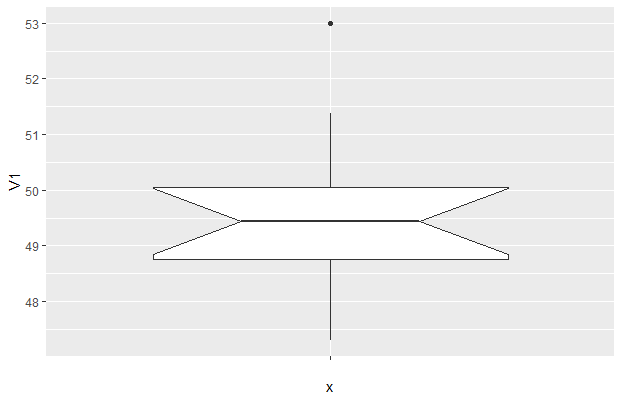##### Share on other sites
• 1

Box plot (box and whisker plot):

This analysis creates visual representation of the range and distribution of Quantitative data (continuous data).

It creates 4 Quartile groups.

Quartile Group 1: Min - 25th Percentile (Q1)
Quartile Group 2: 25th Percentile (Q1) - 50th Percentile (Q2, Median)
Quartile Group 3: 50th Percentile (Q2) - 75th Percentile (Q3)
Quartile Group 4: 75th Percentile (Q3) - Max
In this, Q3-Q1 is Inter Quartile Range (IQR)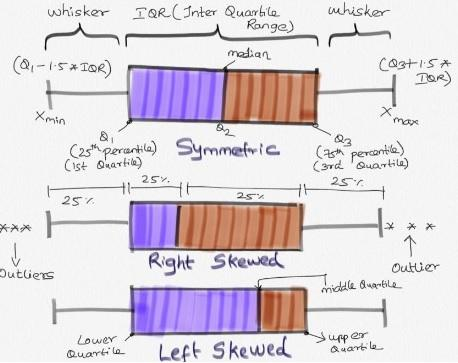Insights from Box-plot:

Comparing multiple data sets (Categorical variable for grouping (1-4);
Understanding Data Symmetry and Skewness

* It gives spread of data points. Lowest(min) and highest(max) value in the data set.
* It shows outliers (if any) present in the data. Outliers are values which is greater than 1.5 times of IQR away from 25th percentile or 75th percentile.
* It clearly shows if the distribution is skewed (left or right. Refer to enclosed pic)
* Median: This separates lower 50% of observations from the upper 50% of observations.
* Box plot with groups, when we have further categories, we can use ‘categorical variables for grouping’, this helps us to identifying further distribution spread among the groups.

Example Reference:

This example is for Box Plot Graph with Groups. Group A and Group B Respectively.

In this, it is clearly evident that there are outliers in both the graph.

Group A is right Skewed. We will have more clarity on the distribution of data in both groups by visual representation.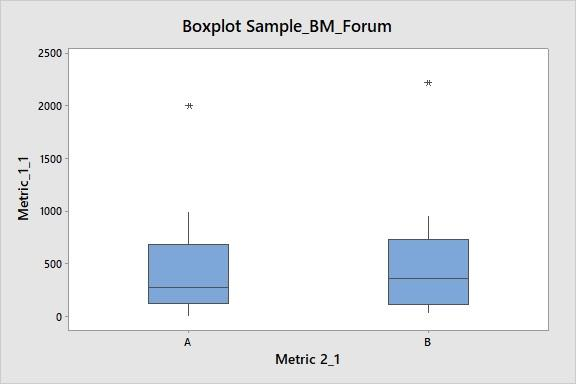##### Share on other sites
• 1

What is Box plot

Is a graphical tool used to know the spread of the data or variability in a set of data.

It also shows whether the distribution having skew or not and outliers means any unusual observation

When to be used

It is to be used when we have large range of data or multiple set of data which is related with each other in some way

It is to be used when predictor value(X) is descrete and respond value (Y)is continuous.

When there is a bias for unusual observation box plot to be used

Example

1.when one wants to show graphical representation of marks scored by students in exam

2.when one wants to show graphical representation of data before and after improvement

3.No of accounts handled by managers,etc

How to interpret the box plot

Box plot has the following main five parts

1.Lower quartile range (Q1): This indicates 25% data belong up to this range

2.Median(Q2): 50% of data points lies below and 50% above this range

3.Upper quartile range(Q3): This indicates 75% data belong up to this range.

4.Minimum value of data set

5.Maximum value of data set

It also indicates whether data has any outlier means the data point much far away from either from Q1 or Q3 calculated by following way

Q1-1.5 X(Q3-Q1)  or Q3+ 1.5X(Q3-Q1)

Line extending from lower end of the box is known as lower whisker and line extending from upper end is known as upper whisker

Just see the examples of average marks obtained in the class

 84 110 98 41 75 60 86 78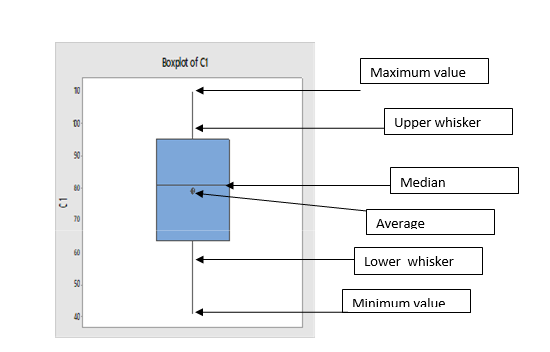We can interpret the graph as below

Minimum score is 40

Maximum score is 110

25% students scored between 40 to 63

50% students scored below 81 and 50% scored above 81

75% students scored between 40 to 95 also we can say 25% students scored above 95

Average marks scored by students is 80

In this way we can easily classify the data by just seeing visually in box plot.

##### Share on other sites
• 1

Visuals are always easy to review and summarize the content. This is precisely the reason a graphical summary is done rather than reading data in multiple rows or columns.

What is a Box Plot?

The most commonly used method for graphical summary is a frequency distribution plot like a histogram (for continuous data). The same data can also be plotted using a box plot which is just another way of looking at the histogram. Box Plot is a top view of the histogram. I took the annual rainfall data (from the GoI website for Andaman and Nicobar) and below is the graphical summary from Minitab.

If you notice, the same data is represented in a histogram and a box plot.

Even though both graphs represent the same data, the two are actually different. I have tried to summarize the differences below

In addition to the insights or usefulness of the Box Plot as captured in the above table, Box Plot can be used in the following scenarios as well

1. Compare data sets for the same metric (I have provided an example below) even when a project is not being done

2. Used to identify the problem in Define phase (too much spread or process shifted to one side)

3. Used to baseline the process performance in Measure phase

4. Used to graphically compare performance of two or more sub-groups (units, departments, centers, shits etc.) in Analyze phase

5. Used to confirm the improvement in the Improve phase (spread will reduce or process is more centered)

6. Check for presence of outliers in data to ensure process control in Control phase

In the below example, I considered the annual rainfall data for 6 regions (from the GoI website).

Observations from the box plot

1. Clearly identifies the regions which get higher rainfall as compared to the others. A&N receive the maximum annual rainfall while Rajasthan West receives the lowest

2. Rainfall in Rajasthan, Delhi, Orissa and UP West (if I ignore the slightly elongated whisker) is almost equally distributed across the range, while it is skewed in A&N (left skewed) and Nagaland (right skewed)

3. The variation in rainfall is the least in Delhi and Rajasthan West while it the max in A&N and Nagaland (given that the length of the box is highest for them)

4. There are no outliers in the data set

Just for illustration, I added another year's data (hypothetically a drought year). Below is how the box plot changes.

Now the box plot, adds an outlier (star mark) for all states except Rajasthan West (i had entered a value of 0, but still it did not consider it as an outlier). These star marks indicate the presence of a value which is different from the other values for the data set or in other words is an outlier. Box Plot identifies it and gives us a chance to investigate and do RCA to find out the reason (remember I had entered data for a hypothetical drought year where rainfall will be very less).

I guess the limitations of Gauss' Normal Distribution Plot and Karl Pearson's Histogram led John Tukey to identify and start using a Box Plot :)

##### Share on other sites
• 0

The specific benefits/ insights obtained by using a Box Plot. Explain with examples

Box Plot is also known as Box & whisker plot.

The key inferential data points are :  Q1, Q3, Inter Quartile Range ( the area between 1st Quartile & 3rd Quartile ), the Whiskers & the very important Outliers. 1st major benefit of Box Plot is the visually identifiable "Outliers" Every Outlier need to be studied to understand if it is a random occurance or if a special cause is associated with it. The Inter Quartile range and the position of the Median explains the spread of the data and a similar understanding of the far out data points can be gained from the length of the whiskers.

Comparing the average rain fall of Top 10 Rainfall supported crop states could be on example while deciding/predicting/recommending which states are likely to have a higher probability of "Good Crop" year.

An Airconditioning brand studying the Average summer temperatures of their Top 25 Markets to arrive at better forcasting of sales for the coming summer.

##### Share on other sites
• 0

Benchmark Six Sigma Expert View by Venugopal R

The table below gives the temperature for XYZ city recorded during 5 different months. For each month 10 readings had been taken randomly across the day.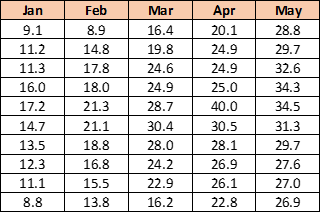If we represent the same data using a Box Plot, it will appear as below. Evidently, the box plot presents the same data in a more easily interpretable manner and mostly self-explanatory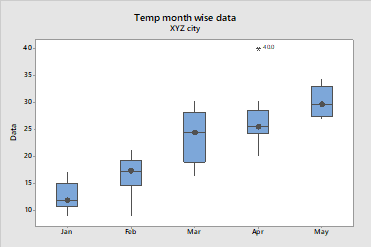The box plot divides the data into 4 quartiles, have median as the measure of central tendency and the height of the box represents the placement of 50% of the data – i.e. between the 1st quartile and the 3rd quartile. Each whisker represents 25% of the data on either end, excluding any outlier. The outliers are shown as a star mark as seen in the above diagram for the month of April. The distance between the 3rd and 1st quartile, or the height of the box is known as ‘Inter Quartile range’. Inter-quartile range is a useful measure of the dispersion, very free from outliers and may be used for comparison between plots.

Thus, the diagrammatic representation of the same data speaks louder, clearer, faster with more elaboration.

##### Share on other sites
• 0In Analyse phase of DMAIC,  Box Plot comes into picture to compare data and analyse properly to display statistics summary at one place for a set of distribution. Box Plot which is also knows as Box and Wishker Plot is another graphical representation of data which can be drawn horizontally and vertically and summarize the following at one place which can be referred in attached picture also:

1. Median

2. Inter - Quartile Range ( Q3-Q1)

3. Quartile range or 25th, 50th, 75th percentile

4. Minimum & Maximum Range of data set

5. Outlier

Interpreting the Box Plot:

Making a Box Plot is easy and very simple but it is very important to know how to interpret it to understand data in a better way which can help us to reach a final conclusion:

1. It consists a Line between the box which is called median or 50th Percentile or Q2

2. The Lower Side of The Box Plot is Q1 or 25th Percentile

3. The Upper Side of the Box Plot is Q3 or 75th Percentile

4. 50% data is contained by Box Plot Itself as the Length of The Box is Q3-Q1

5. The ends of the vertical straight lines represent the Smallest and Largest observed Data Value

6. in picture outlier is shown by circle, normally it is represented by dot or asterisk mark.

7. If the median line is not in the center it means the data is skewed.

Despite the simplicity of Box Plot, it is very beneficial and contains a lot of useful information which can be seen here without using much more statistical tools. Following are the benefits of Box Plot:

1. Median can be used to determine central tendency or location

2. With the help of Box Length we can determine the spread, variability.

3. with the help of outlier we get to know about outliers in the data

4. Skewness, symmetry of the data can be identified with the help of median location.

5. if the median is laying towards bottom of box then the data is positively skewed.

6. if the median is laying towards top  of the box then the data is negatively skewed.

7. On the same graph box plot can be used to compare different data sets.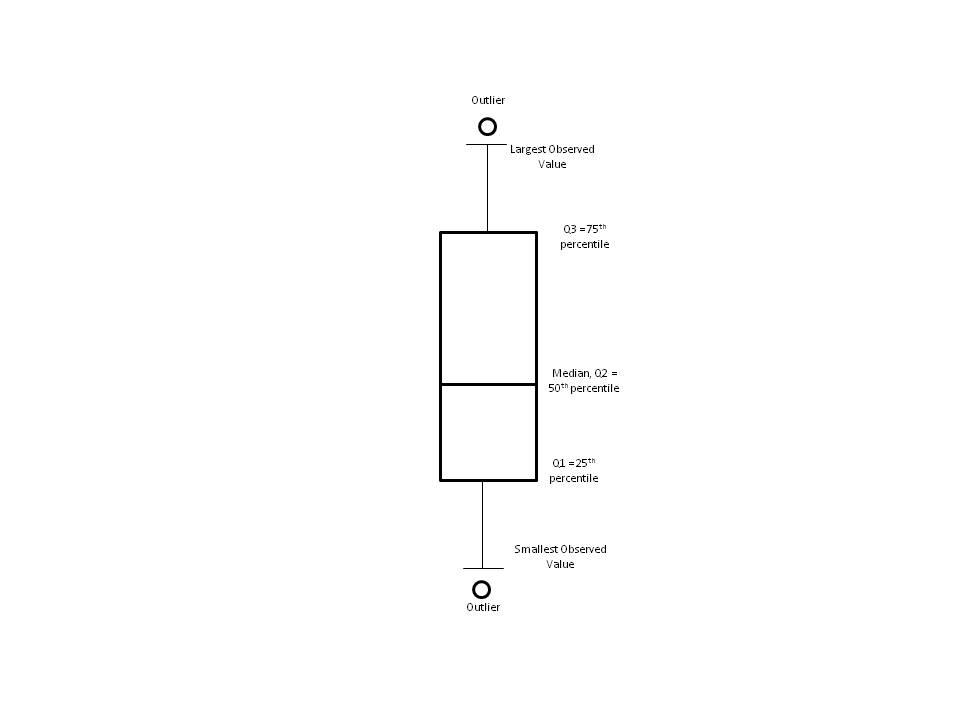##### Share on other sites
• 0

1. It will graphically displays a variables location & spread at a glance.

2.provides some indication of the datas symmetry and skewness.

3. Boxplot shows the outliers.

4. One can quickly compare the data sets by using boxplots

Example  - Histogram.

##### Share on other sites
• 0
On 6/28/2019 at 2:18 PM, Vishwadeep Khatri said:

Explanation: Box plot helps use to understand the spread of the data by graphically representing the outliers and based on the nature of the business, either one can choose to identify the RCA or else can remove the outlier from the data before moving towards the next phase of analysing the trend with the help of other tools and techniques since as a disadvantage of box plot, it has no relevance when one has to to analysis the data in detail.

Example: As in my last organisation, each quality auditor has to do a certain count of audits each day and also have a monthly target. Every QA was able to complete their monthly targets however when we analyzed the data using boxplot to understand how team was completing target. It was seen that few QA's were irregular in completing their daily audit counts and as a result they were auditing more than daily target audit count on a single day.
The rule of Quality says that each individual has a capacity to barge a certain amount of calls to deliver best result and if the same person will audit multiple calls on single day, the probability of delivering will be impacted.
Hence, while studying the performance graph we excluded the bulk audits by these QA's and were able to drive actual critical X's from the quality parameters.
Also, as per business call, we planned time management activities for the outliers (identified QA's) to improve the overall process.

##### Share on other sites
• 0

Box Plot is one of the most effective and efficient ways of representing the data. It is one of the standardized ways of representing the data in the form of different Quartiles.

The box plot helps distinctly in following important ways:

1. Helps in understanding the outliers in the group.

2. Clear visual representation and standardization of the data, hence facilitating the decision making process.

3.Helps the leaders to understand the pattern and behaviour of data.

4.Minimum, Maximum and Median can be understood clearly unambiguously.

5.Spread of the data can be understood in simple and clear manner.

For example, the performance of the different  sets of vendors in the process of service deliver, classification of students in a class to score marks, performance of machine at varying indefinite variable parameters, etc.

Moreover, the BOX PLOT also helps to determine the Interquartile range i.e., Q1-Q3, This helps to compare the distribution of data into different segments. Box plot is more of a analytical and decision making statistical tool that represents the data in  more striuctured, standardized manner.

##### Share on other sites
• 0

Thank you for a phenomenal response to the question. All the respondents have brought forth important aspects about the Boxplot.  There are 3 winners today:

Amlan - for explaining where the 1.5 IQR length concept came from and also mentioning situations where a Boxplot fails, besides using pictures to explain, where needed.

Mohammed Asif - for well structured answer with pictures and an example of how Boxplot can be used for subgroup comparison

Natwar Lal - for adding a twist to the answer and building up the Boxplot concept from a Histogram and sharing examples.

(In no particular order) Rachit has shared a simple practical example on how Boxplot can be used to drive improvements, Nilesh and Vastu's exaplanations are simple to read and understand for complete beginners.

##### Share on other sitesThis topic is now closed to further replies.
• ### Who's Online (See full list)

There are no registered users currently online

• ### Forum Statistics

• Total Topics
2,877
• Total Posts
14,579
• ### Member Statistics

• Total Members
55,093
• Most Online
888